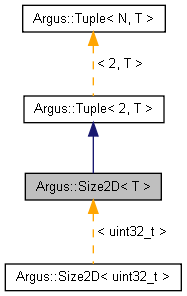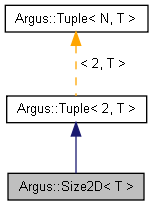# Jetson Linux API Reference

##### 32.7.1 Release
Argus::Size2D< T > Class Template Reference

## Detailed Description

### template<typename T> class Argus::Size2D< T >

Size2D template class.

This is a Tuple specialization containing 2 elements corresponding to the width and height of a 2D size, in that order. Values can be accessed using the named methods.

Definition at line 705 of file Types.h.

Inheritance diagram for Argus::Size2D< T >:[legend]
Collaboration diagram for Argus::Size2D< T >:[legend]

## Public Member Functions

Size2D ()

Size2D (const Tuple< 2, T > &other)

Size2D (T init)

Size2D (T _width, T _height)

T & width ()

const T & width () const

T & height ()

const T & height () const

area () const
Returns the area of the size (width * height). More...

bool operator== (const Tuple< N, T > &rhs) const
Returns true when every element in the two tuples are identical. More...

bool operator!= (const Tuple< N, T > &rhs) const
Returns true if there are any differences between the two tuples. More...

Tuple< N, T > & operator+= (const Tuple< N, T > &rhs)
Adds every element of another tuple to the elements of this tuple. More...

Tuple< N, T > & operator-= (const Tuple< N, T > &rhs)
Subtracts every element of another tuple from the elements of this tuple. More...

Tuple< N, T > & operator*= (const T &rhs)
Multiplies every element in the tuple by a single value. More...

Tuple< N, T > & operator/= (const T &rhs)
Divides every element in the tuple by a single value. More...

const Tuple< N, T > operator+ (const Tuple< N, T > &rhs) const
Returns the result of adding another tuple to this tuple. More...

const Tuple< N, T > operator- (const Tuple< N, T > &rhs) const
Returns the result of subtracting another tuple from this tuple. More...

const Tuple< N, T > operator* (const T &rhs) const
Returns the result of multiplying this tuple by a single value. More...

const Tuple< N, T > operator/ (const T &rhs) const
Returns the result of dividing this tuple by a single value. More...

T & operator[] (unsigned int i)

const T & operator[] (unsigned int i) const

## Static Public Member Functions

static unsigned int tupleSize ()
Returns the number of elements in the tuple. More...

m_data [N]

## ◆ Size2D() [1/4]

template<typename T >
 Argus::Size2D< T >::Size2D ( )
inline

Definition at line 708 of file Types.h.

## ◆ Size2D() [2/4]

template<typename T >
 Argus::Size2D< T >::Size2D ( const Tuple< 2, T > & other )
inline

Definition at line 709 of file Types.h.

## ◆ Size2D() [3/4]

template<typename T >
 Argus::Size2D< T >::Size2D ( T init )
inline

Definition at line 711 of file Types.h.

## ◆ Size2D() [4/4]

template<typename T >
 Argus::Size2D< T >::Size2D ( T _width, T _height )
inline

Definition at line 716 of file Types.h.

## ◆ area()

template<typename T >
 T Argus::Size2D< T >::area ( ) const
inline

Returns the area of the size (width * height).

Definition at line 728 of file Types.h.

## ◆ height() [1/2]

template<typename T >
 T& Argus::Size2D< T >::height ( )
inline

Definition at line 724 of file Types.h.

## ◆ height() [2/2]

template<typename T >
 const T& Argus::Size2D< T >::height ( ) const
inline

Definition at line 725 of file Types.h.

## ◆ operator!=()

 bool Argus::Tuple< N, T >::operator!= ( const Tuple< N, T > & rhs ) const
inlineinherited

Returns true if there are any differences between the two tuples.

Definition at line 500 of file Types.h.

## ◆ operator*()

 const Tuple Argus::Tuple< N, T >::operator* ( const T & rhs ) const
inlineinherited

Returns the result of multiplying this tuple by a single value.

Definition at line 550 of file Types.h.

## ◆ operator*=()

 Tuple& Argus::Tuple< N, T >::operator*= ( const T & rhs )
inlineinherited

Multiplies every element in the tuple by a single value.

Definition at line 522 of file Types.h.

## ◆ operator+()

 const Tuple Argus::Tuple< N, T >::operator+ ( const Tuple< N, T > & rhs ) const
inlineinherited

Returns the result of adding another tuple to this tuple.

Definition at line 538 of file Types.h.

## ◆ operator+=()

 Tuple& Argus::Tuple< N, T >::operator+= ( const Tuple< N, T > & rhs )
inlineinherited

Adds every element of another tuple to the elements of this tuple.

Definition at line 506 of file Types.h.

## ◆ operator-()

 const Tuple Argus::Tuple< N, T >::operator- ( const Tuple< N, T > & rhs ) const
inlineinherited

Returns the result of subtracting another tuple from this tuple.

Definition at line 544 of file Types.h.

## ◆ operator-=()

 Tuple& Argus::Tuple< N, T >::operator-= ( const Tuple< N, T > & rhs )
inlineinherited

Subtracts every element of another tuple from the elements of this tuple.

Definition at line 514 of file Types.h.

## ◆ operator/()

 const Tuple Argus::Tuple< N, T >::operator/ ( const T & rhs ) const
inlineinherited

Returns the result of dividing this tuple by a single value.

Definition at line 556 of file Types.h.

## ◆ operator/=()

 Tuple& Argus::Tuple< N, T >::operator/= ( const T & rhs )
inlineinherited

Divides every element in the tuple by a single value.

Definition at line 530 of file Types.h.

## ◆ operator==()

 bool Argus::Tuple< N, T >::operator== ( const Tuple< N, T > & rhs ) const
inlineinherited

Returns true when every element in the two tuples are identical.

Definition at line 494 of file Types.h.

## ◆ operator[]() [1/2]

 T& Argus::Tuple< N, T >::operator[] ( unsigned int i )
inlineinherited

Definition at line 561 of file Types.h.

## ◆ operator[]() [2/2]

 const T& Argus::Tuple< N, T >::operator[] ( unsigned int i ) const
inlineinherited

Definition at line 562 of file Types.h.

## ◆ tupleSize()

 static unsigned int Argus::Tuple< N, T >::tupleSize ( )
inlinestaticinherited

Returns the number of elements in the tuple.

Definition at line 565 of file Types.h.

## ◆ width() [1/2]

template<typename T >
 T& Argus::Size2D< T >::width ( )
inline

Definition at line 722 of file Types.h.

## ◆ width() [2/2]

template<typename T >
 const T& Argus::Size2D< T >::width ( ) const
inline

Definition at line 723 of file Types.h.

## ◆ m_data

 T Argus::Tuple< N, T >::m_data[N]
protectedinherited

Definition at line 568 of file Types.h.

The documentation for this class was generated from the following file: